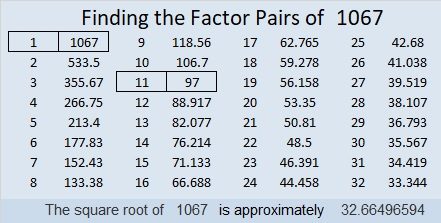# 1067 and Level 5

The common factors of 20 and 40 are 1, 2, 4, 5, and 10. Only the ones in blue will put numbers from 1 to 12 in the top row, as required. Since there is more than one possible common factor, don’t start with those two clues. This is a level 5 puzzle so at least one pair of clues will work to get you started.Print the puzzles or type the solution in this excel file: 12 factors 1063-1072

Here are a few facts about the number 1067:

• 1067 is a composite number.
• Prime factorization: 1067 = 11 × 97
• The exponents in the prime factorization are 1 and 1. Adding one to each and multiplying we get (1 + 1)(1 + 1) = 2 × 2 = 4. Therefore 1067 has exactly 4 factors.
• Factors of 1067: 1, 11, 97, 1067
• Factor pairs: 1067 = 1 × 1067 or 11 × 97
• 1067 has no square factors that allow its square root to be simplified. √1067 ≈ 32.664971067 is the hypotenuse of a Pythagorean triple:
715-792-1067 which is 11 times (65-72-97)
We can use the 11 divisibility trick on all the numbers in that triple:
7 – 1 + 5 = 11
7 – 9 + 2 = 0
1 – 0 + 6 – 7 = 0
to see that all of them can indeed be evenly divided by 11.

1067 is palindrome 1F1 in BASE 26 (F is 15 base 10) because 26² + 15(26) + 1 = 1067